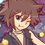# Can you solve these Number Theory problems in 30 seconds & 1 minute?

If possible, list all the solutions in limited time too!

You have 30 seconds for solving these problems.

Def: Lattice point is the point $(x,y)$ such that both $x$ and $y$ are integers.

1.) Find the number of lattice points of a hyperbola given by an equation

$4x^{2}-y^{2}-2y-2558 = 0$

2.) Find the number of lattice points of an ellipse given by an equation

$2x^{2}-xy+2y^{2}+3x-3y-3 = 0$

You have 1 minute for solving these problems.

1.) Find the number of ordered pair $(x,y)$ in integers of the equation

$x^{2} - y! = 2559$

2.) Find the number of ordered triples $(x,y,z)$ in integers of the equation

$x^{2}+y^{2}+z^{2} = 2558xyz$

Sometimes the math competitions in my country are too goddamn crazy. I hate it, and I'll never have this competition again. XDNote by Samuraiwarm Tsunayoshi
5 years, 5 months ago

This discussion board is a place to discuss our Daily Challenges and the math and science related to those challenges. Explanations are more than just a solution — they should explain the steps and thinking strategies that you used to obtain the solution. Comments should further the discussion of math and science.

When posting on Brilliant:

• Use the emojis to react to an explanation, whether you're congratulating a job well done , or just really confused .
• Ask specific questions about the challenge or the steps in somebody's explanation. Well-posed questions can add a lot to the discussion, but posting "I don't understand!" doesn't help anyone.
• Try to contribute something new to the discussion, whether it is an extension, generalization or other idea related to the challenge.

MarkdownAppears as
*italics* or _italics_ italics
**bold** or __bold__ bold
- bulleted- list
• bulleted
• list
1. numbered2. list
1. numbered
2. list
Note: you must add a full line of space before and after lists for them to show up correctly
paragraph 1paragraph 2

paragraph 1

paragraph 2

[example link](https://brilliant.org)example link
> This is a quote
This is a quote
    # I indented these lines
# 4 spaces, and now they show
# up as a code block.

print "hello world"
# I indented these lines
# 4 spaces, and now they show
# up as a code block.

print "hello world"
MathAppears as
Remember to wrap math in $$ ... $$ or $ ... $ to ensure proper formatting.
2 \times 3 $2 \times 3$
2^{34} $2^{34}$
a_{i-1} $a_{i-1}$
\frac{2}{3} $\frac{2}{3}$
\sqrt{2} $\sqrt{2}$
\sum_{i=1}^3 $\sum_{i=1}^3$
\sin \theta $\sin \theta$
\boxed{123} $\boxed{123}$

Sort by:

I just might say I can.

For the first question, we change it to $4{ x }^{ 2 }-{ (y+1) }^{ 2 }=2557$, and as $4x^2$ is divisible by 4, $4{ x }^{ 2 }-{ (y+1) }^{ 2 }$ will have a remainder of 0 or -1 when divided by 4, which is not the case here.

Next question, multiply both sides by 2, then we will have ${ (x-y) }^{ 2 }+{ 3(x+1) }^{ 2 }+{ 3(y-1) }^{ 2 }=12$, and we can then claim that ${ (x-y) }^{ 2 }$ must be 0 or 9 (as 12 and ${ 3(x+1) }^{ 2 }+{ 3(y-1) }^{ 2 }$ are all divisible by 3). The last part is pretty lengthy, so I will not write it down here (besides, it's easy from here)

The third one, we have ${ x }^{ 2 }=2559+y!$, which means $x^2$ will have the remainder of 3 when divided by 4 if $y \ge 4$, which is impossible. Test for numbers from 1 to 3, tada, we have the answer.

The final one, if none of the 3 is divisible by 2 then $x^2+y^2+z^2$ is not divisible by 2 while $2558xyz$ is not. If one or two is then $x^2+y^2+z^2$ is not divisible by 4 while $2558xyz$ is, which means that all 3 must be divisible by 2. Let ${x}^{'}=\frac{x}{2},{y}^{'}=\frac{y}{2},{z}^{'}=\frac{z}{2}$ and prove exactly like shown above. Also note that if $x,y,z$ are positive integers, then there does not exist a set of infinitely many numbers $x_1,...x_n$ which satisfies $x_1>x_2>...>x_n$, which leaves $x=y=z=0$ the only solution.

The first 2 problems I solved within 20 seconds each, while the third was solved in 10 seconds and the final one took me almost a minute. I think I should start celebrating now.

- 2 years, 10 months ago

Are you really 14 years old?

- 2 years, 3 months ago

I'm sure 10th graders are 14-15 years old, so yep, you're right.

Just a math lover :)

P/S: Not bragging, but these are still easy to what we have to do :) If you really want to screw your brain up, I have some for you :D

- 2 years, 3 months ago

I am in grade 10 and 99% of my classmates don't even know the quadratic equation or factorials. They only know BIDMAS and cubic graphs nothing else special.... hence I look like a genius at my class but thanks to Brilliant; I identified that it's the school's fault of having such low mathematic standards and that globally i am literally somewhat a zero hero :(

However, due to Brilliant; I am able to increase my standard and understanding of mathematics in a dramatic way.

:D

- 2 years, 3 months ago